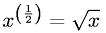Equations > Algebra > Exponents/Powers > Definition of Square Root

### Definition of Square RootLatex Code:

MathML Code:

 ${x}^{\left(\frac12\right)}=\sqrt{x}$

MathType 5.0: